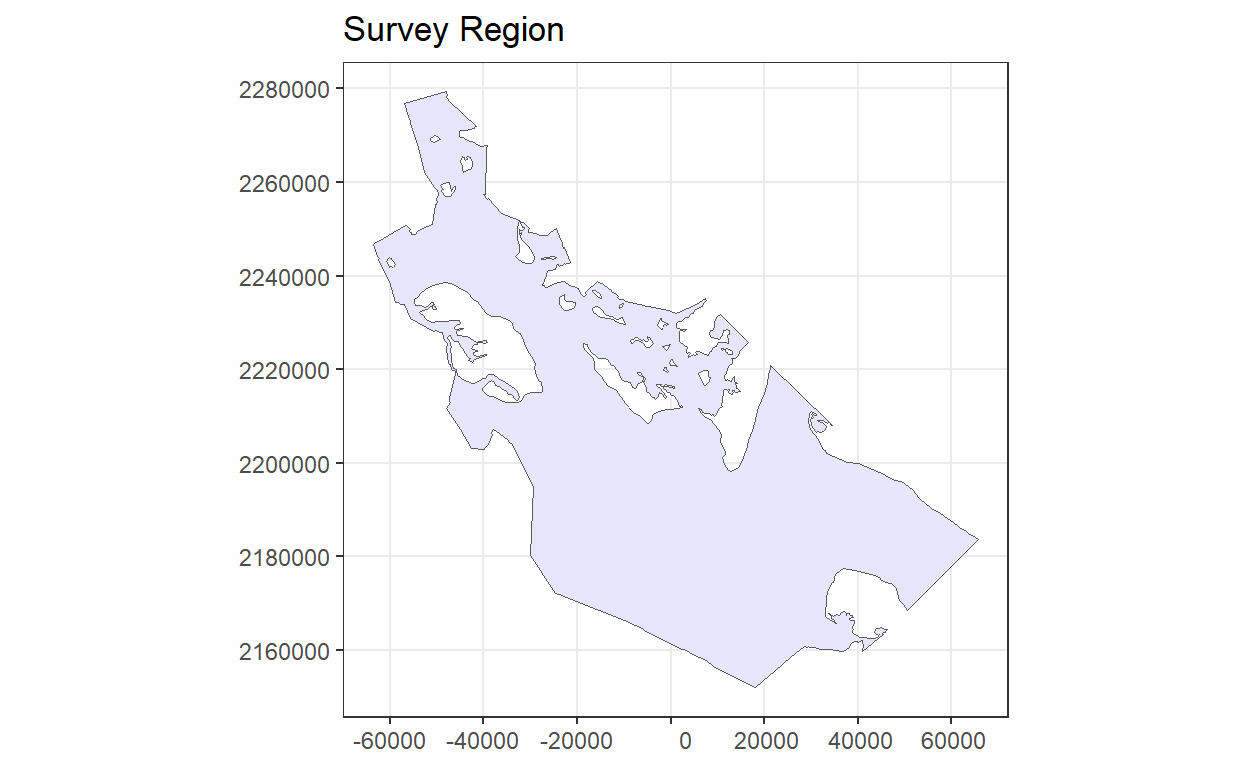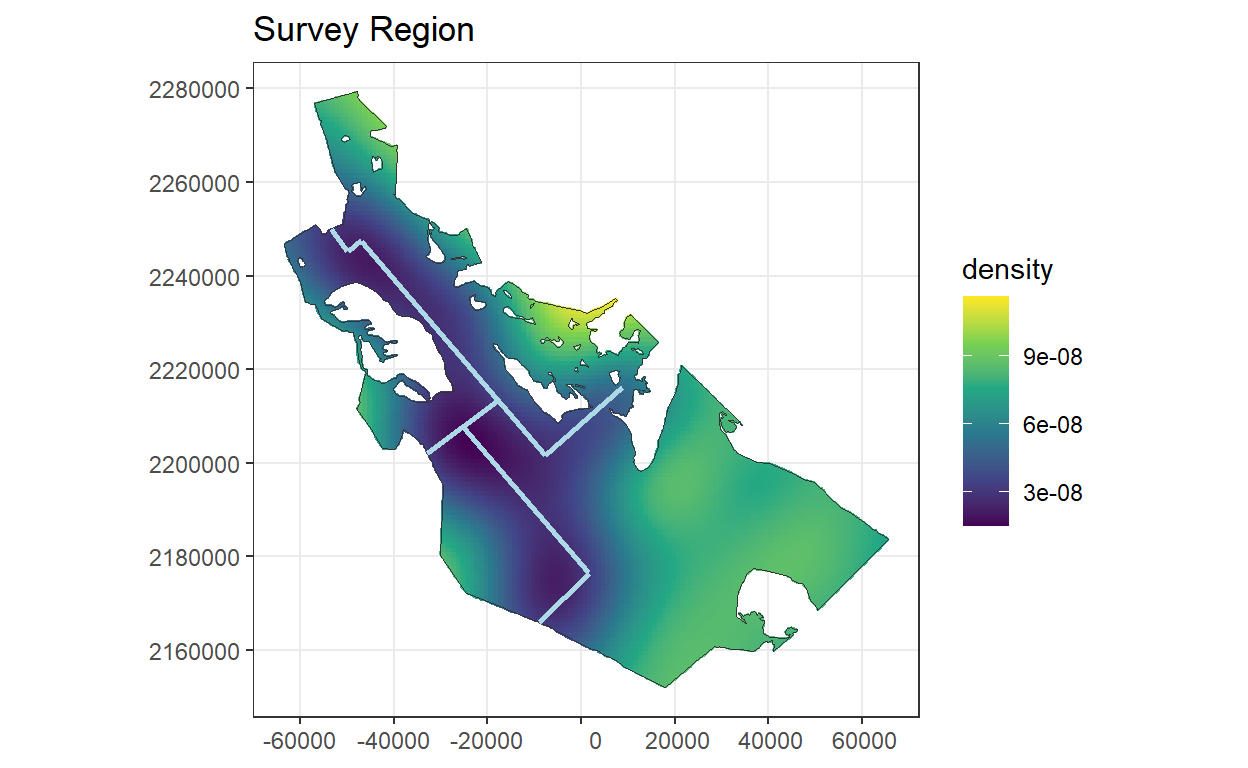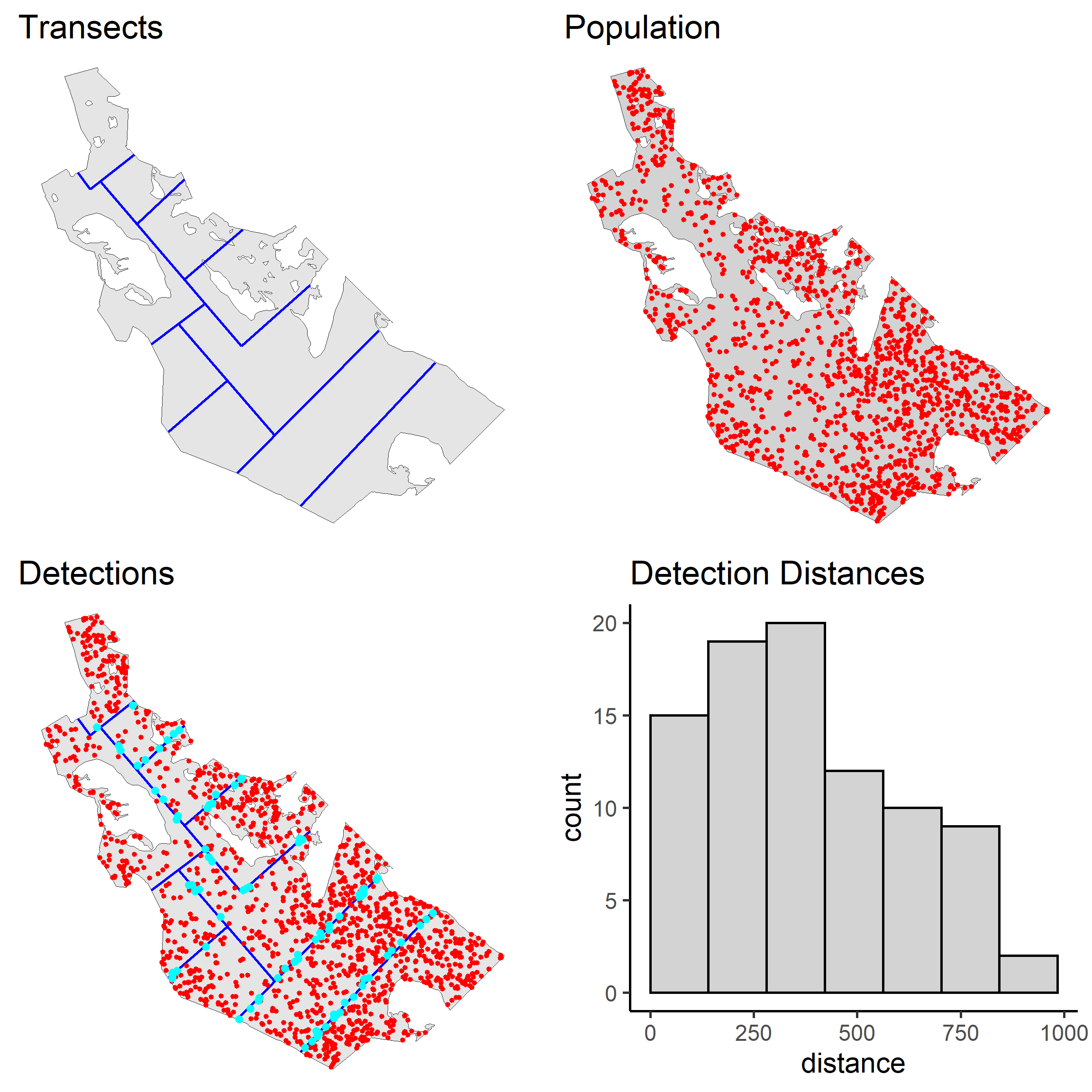# Distance sampling simulation included in Buckland et al. (2015)

Description and R code to produce simulation described in Sections 3.5.2 and 11.1.1 in Buckland et al. (2015); demonstrating the use of the package dsims to evaluate subjective vs random placement of transects. Simulation designed and implemented by L. Marshall.

Laura Marshall http://distancesampling.org (CREEM, Univ of St Andrews)https://creem.st-andrews.ac.uk
2022-09-27
library(knitr)
library(dsims)

This case study shows you how to use the R package dsims to compare the performance of different survey designs. We will replicate the analyses of Sections 2.5.2 and 11.1.4 of . These simulations compare the efficiency of the randomised systematic parallel and equal spaced zigzag designs as well as demonstrating how easily bias can be introduced when non-random subjective designs are used.

# Getting started

Ensure you have administrator privileges on your computer and install the necessary R packages.

needed.packages <- c("dsims")
myrepo <- "http://cran.rstudio.com"
install.packages(needed.packages, repos = myrepo)

## Directory structure for files in this project

In addition to the R packages, there are additional files required by this analysis. All necessary material are included in a Zip archive file entitled dsims_study.zip. When that archive is uncompressed, the directory structure will be created as described.

Examine the other files and folders in the “dsims_study” folder. There are three files starting with the name “Region” and ending with .dbf, .shp and .shx, these files make up the shapefile for the survey region. The density.robj file is the density surface for the survey region. The Survey Transects folder contains files wth the name “subj_des” describing the shapefile to be used for the subjective design. The Results folder contains the results from 999 replications as this can take a good few hours to run. Select the directory that contains these files.

# Creating a new simulation

## Creating a region object

First we create the region object using the region shapefile. As there are no strata in this example, you just need to provide a name for your survey region and specify the units (metres, m) as there is no projection information supplied with this shapefile.

region <- make.region(region.name = "Survey Region", units = "m", shape = "Region.shp")

View the resulting object:

plot(region)## Creating a density object

Now create a density object within this region. For this study, a density surface has already been created, but you can experiment with the options in the next code chunk to define one yourself.

You can create other density surfaces by creating a density object based on a uniform density grid over the area, and adding some hot spots (or low spots).

density <- make.density(region = region, x.space = 1000, y.space = 1000,
constant = 4e-08)
density <- add.hotspot(density, centre = c(-2500, 2224000), sigma = 10000,
amplitude = 1e-08)
density <- add.hotspot(density, centre = c(0, 2184000), sigma = 18000,
amplitude = -5e-09)
# Plot this example density surface
plot(density, region)

Load the predefined density object and view the data that comprise the surface by plotting it. This density surface was created using a combination of adding low and high spots and also calculating density as a function of distance to path (the paths are shown on the density plot in light blue). The values obtained from this process were then smoothed by fitting a generalised additive model and predicting to the density grid points.

load("density.robj")
# Save the ggplot object rather than displaying automatically
density.plot <- plot(density, region)

library(sf)
library(ggplot2)
paths <- sf::st_read("Survey_Transects/Paths.shp", quiet = TRUE)
density.plot + ggplot2::geom_sf(data = paths, mapping = aes(), colour = "light blue",
lwd = 1)### Population size

Fix the population size at 1500 individuals. Using make.population.description set the first argument to the desired population abundance and set the second argument such that exactly this number of individuals is generated in each realisation (fixed.N = TRUE).

pop.description <- make.population.description(region = region, density = density,
N = 1500, fixed.N = TRUE)

### True detection function

We select a half-normal detection function with a $$\sigma$$ (scale.param) of 500m and a truncation distance of 1000m.

detect <- make.detectability(key.function = "hn", scale.param = 500, truncation = 1000)

## Creating the survey design object

We first consider the subjective design (Section 11.1.4 of ). The subjective design uses existing paths together with additional transects chosen to achieve a more even coverage of the survey region.

There is no specific definition in dsims (in fact the design package dssd ) for subjective designs but we can instead define it as a random line transect design. When we run the survey or simulation we will then point to the shapefile containing the transects that we wish to use.

subjective.design <- make.design(transect.type = "line", design = c("random"),
region = region, edge.protocol = "minus", truncation = 1000)

## Creating the analyses object

Describe the analyses to carry out on the simulated data. Here we propose both half-normal and hazard-rate models (neither with covariates) and choose between them based on the AIC values.

ds.analyses <- make.ds.analysis(dfmodel = list(~1, ~1), key = c("hn", "hr"),
truncation = 1000, criteria = "AIC")

## Creating the simulation object

We can finally put it all together and have a look at some example populations, transects and survey data. Set the number of repetitions (reps) to be fairly low initially to avoid long run times.

simulation.subjective <- make.simulation(reps = 10, design = subjective.design,
population.description = pop.description, detectability = detect, ds.analysis = ds.analyses)

Before running the simulation, you can check to see that it is doing what you want by using the run.survey function to create an instance of a single simulated survey based on the simulation setup. At this point given that we want to use a set of pre-created transects we will need to specify the shapefile to use.

# Get the
transect.filename <- paste(getwd(), "/Survey_Transects/Subj_Des.shp", sep = "")
# Simulate a single survey
survey <- run.survey(simulation.subjective, filename = transect.filename)

plot(survey, region)If the previous plots lead you to believe you have properly parameterised your simulation, it is time to run it. Be patient, as it will take a few minutes to complete, even though there are only 10 replicates. Again we need to supply the path to our subjective design transects for the simulation (otherwise it will generate randomly placed lines).

simulation.subjective.run <- run.simulation(simulation.subjective, transect.path = transect.filename)

Information about effort, estimated abundance, estimated density and detection probability $$(P_a)$$ is available in the resulting object. Results from each replicate for abundance estimation can be examined, such as

kable(t(simulation.subjective.run@results$individuals$N[, , 5]), digits = 3,
caption = "Estimate of abundance (with measures of precision) for the fifth replicate of the simulation performed above.")
Table 1: Estimate of abundance (with measures of precision) for the fifth replicate of the simulation performed above.
Estimate se cv lcl ucl df
967.129 215.663 0.223 606.767 1541.511 16.351

or average abundance estimates across replicates

kable(t(simulation.subjective.run@results$individuals$N[, , 11]), digits = 3,
caption = "Average estimate of abundance (with measures of precision) across all replicates of the simulation performed above.")
Table 2: Average estimate of abundance (with measures of precision) across all replicates of the simulation performed above.
Estimate se cv lcl ucl df
1188.555 286.616 0.242 716.91 1985.6 14.435

There is also a summary() function for simulation results objects that provide more exhaustive results.

# On to randomised designs

You will need to create 2 new simulations each with a new design object, one for the parallel design and one for the zigzag design. The spacings of these designs were chosen so that the cyclic line lengths were similar, this would imply that they would have similar costs to complete. The other objects (region, density, population description etc.) should not be changed. Here is how to do it for the parallel design. As these randomised designs can be generated automatically in R using the dssd package (via dsims), we no longer need to supply a the shapefile(s) when we run the survey or simulation.

parallel.design <- make.design(transect.type = "line", design = "systematic",
region = region, design.angle = 45, spacing = 12000, edge.protocol = "minus",
truncation = 1000)

simulation.parallel <- make.simulation(reps = 999, design = parallel.design,
population.description = pop.description, detectability = detect, ds.analysis = ds.analyses)

The code does not complete the process of analysing and summarising the simulation of systematic parallel line transects. The user can complete this investigation. The parameterisation of the zigzag design should be set up as shown below.

zigzag.design <- make.design(transect.type = "line", design = "eszigzag",
region = region, design.angle = 135, spacing = 8250, bounding.shape = "convex.hull",
edge.protocol = "minus", truncation = 1000)

simulation.zigzag <- make.simulation(reps = 999, design = zigzag.design,
population.description = pop.description, detectability = detect, ds.analysis = ds.analyses)

## Contrast bias and precision from subjective and randomised surveys

More than 10 replicates are needed to adequately address the question of bias. Results of a more extensive simulation study are stored as workspace objects. The following code loads these results.

load("Results/simulation_subjective.robj")
load("Results/simulation_zigzag.robj")

We can extract components from these R objects to approximate the findings presented in Table 2.1, page 29 of .

extract.metrics <- function(simobj) {
nreps <- simobj@reps
data <- simobj@results$individuals$summary
effort.avg <- data[, , nreps + 1]/1000
detects.avg <- data[, , nreps + 1]
abund <- simobj@results$individuals$N
abund.avg <- abund[, , nreps + 1]
true.N <- simobj@population.description@N
est.bias <- (abund.avg - true.N)/true.N
est.bias.pct <- est.bias * 100
mean.stderr <- abund[, , nreps + 1]
stddev <- abund[, , nreps + 2]
result.vector <- unname(c(effort.avg, detects.avg, abund.avg, est.bias.pct,
mean.stderr, stddev))
return(result.vector)
}
subjective <- extract.metrics(simulation.subjective)
parallel <- extract.metrics(simulation.parallel)
zigzag <- extract.metrics(simulation.zigzag)

result.table <- data.frame(subjective, parallel, zigzag, row.names = c("Mean effort(km)",
"Mean sample size", "Mean abundance estimate", "Estimated percent bias",
"Mean SE of abundance estimate", "SD of abundance estimates"))
kable(result.table, digits = 1, caption = "Simulation results summary for each design.  Estimates based upon 999 simulations with true abundance of 1500 objects.")
Table 3: Simulation results summary for each design. Estimates based upon 999 simulations with true abundance of 1500 objects.
subjective parallel zigzag
Mean effort(km) 339.8 473.2 694.5
Mean sample size 87.2 145.9 215.1
Mean abundance estimate 1225.1 1477.5 1483.8
Estimated percent bias -18.3 -1.5 -1.1
Mean SE of abundance estimate 270.7 206.3 172.7
SD of abundance estimates 208.9 198.5 155.4

## Discussion

The subjective design is included to demonstrate how easily bias can be introduced using non-random designs. One may have been reassured that the animals did not have a significant response to the paths given that there were a number of detections made along the paths, however including the paths in the survey led to a negative bias of around 18%.

The systematic parallel design and the equal spaced zigzag design demonstrate the potential trade off between accuracy and precision. These two particular designs both gave cyclic trackline lengths of 845 km indicating they would be of similar cost to complete (assuming on-effort surveying is no more costly than off-effort travel). Systematic parallel line designs give even coverage across the majority of the study region (with the exception of the very edge when minus sampling is used and coverage is slightly lower here). Zigzag designs can be more efficient in terms of maximising the amount of time spent on-effort surveying and therefore can increase precision, however they can also give non-uniform coverage which may potentially lead to bias. In this example, there is very low bias in both designs but we see an improvement in precision for the zigzag design. The results of such comparisons will vary for each individual survey depending on the degree of non-uniform coverage generated by the zigzag design and the distribution of animals within the survey region.

Buckland, S. T., Rexstad, E. A., Marques, T. A., & Oedekoven, C. S. (2015). Distance sampling: Methods and applications. Retrieved from https://www.springer.com/gp/book/9783319192185